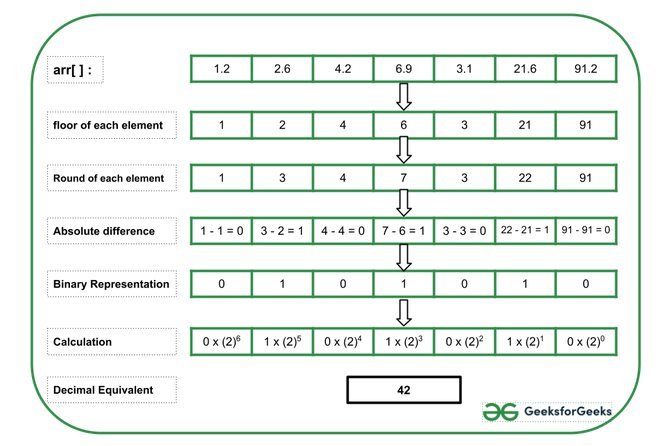GeeksforGeeks App
Open AppBrowser
Continue

# Decimal equivalent of concatenation of absolute difference of floor and rounded-off values of array elements as a Binary String

Given an array arr[] consisting of N floating-point numbers, the task is to print the decimal representation of the binary array constructed from the absolute difference between the floor and round-off value for each array element.

Examples:

Input: arr[] = {1.2, 2.6, 4.2, 6.9, 3.1, 21.6, 91.2}
Output: 42
Explanation:
Below is the image to illustrate the above example:Input: arr[] = {5.7, 2.8, 1.9, 5.6, 2.2}
Output: 30

Approach: Follow the steps below to solve the problem:

• Initialize a variable, say result as 0 that stores the resultant numbers formed.
• Initialize a variable, say power as 0 that keeps the power of 2 added in each step.
• Traverse the given array arr[] from the end and perform the following steps:
• Initialize a variable, say bit that stores the absolute difference between the round-off value and the floor value of each array element.
• If the value of the absolute difference is 1, then multiply the digit with the proper power of 2 and add it to the variable result.
• Increment the value of power by 1.
• After completing the above steps, print the value of the result as the required decimal equivalent of the binary representation.

Below is the implementation of the above approach:

## C++

 `// C++ program for the above approach` `#include ``using` `namespace` `std;` `// Function to find the decimal equivalent``// of the new binary array constructed``// from absolute decimal of floor and``// the round-off values``int` `findDecimal(``float` `arr[], ``int` `N)``{``    ``int` `bit, power = 0, result = 0;` `    ``// Traverse the givenarray from``    ``// the end``    ``for` `(``int` `i = N - 1; i >= 0; i--) {` `        ``// Stores the absolute difference``        ``// between floor and round-off``        ``// each array element``        ``bit = ``abs``(``floor``(arr[i])``                  ``- round(arr[i]));` `        ``// If bit / difference is 1, then``        ``// calculate the bit by proper``        ``// power of 2 and add it to result``        ``if` `(bit)``            ``result += ``pow``(2, power);` `        ``// Increment the value of power``        ``power++;``    ``}` `    ``// Print the result``    ``cout << result;``}` `// Driver Code``int` `main()``{``    ``float` `arr[] = { 1.2, 2.6, 4.2, 6.9,``                    ``3.1, 21.6, 91.2 };``    ``int` `N = ``sizeof``(arr) / ``sizeof``(arr);``    ``findDecimal(arr, N);` `    ``return` `0;``}`

## Java

 `// Java program for above approach``import` `java.io.*;``import` `java.lang.*;``import` `java.util.*;` `class` `GFG``{` `// Function to find the decimal equivalent``// of the new binary array constructed``// from absolute decimal of floor and``// the round-off values``static` `void` `findDecimal(``double` `arr[], ``int` `N)``{``    ``int` `bit, power = ``0``, result = ``0``;``  ` `    ``// Traverse the givenarray from``    ``// the end``    ``for` `(``int` `i = N - ``1``; i >= ``0``; i--)``    ``{``  ` `        ``// Stores the absolute difference``        ``// between floor and round-off``        ``// each array element``        ``bit = Math.abs((``int``)Math.floor(arr[i])``                  ``- (``int``)Math.round(arr[i]));``  ` `        ``// If bit / difference is 1, then``        ``// calculate the bit by proper``        ``// power of 2 and add it to result``        ``if` `(bit != ``0``)``            ``result += Math.pow(``2``, power);``  ` `        ``// Increment the value of power``        ``power++;``    ``}``  ` `    ``// Print the result``    ``System.out.print(result);``}` `    ``// Driver Code``    ``public` `static` `void` `main(String[] args)``    ``{``    ``double` `arr[] = { ``1.2``, ``2.6``, ``4.2``, ``6.9``,``                    ``3.1``, ``21.6``, ``91.2` `};``    ``int` `N = arr.length;``    ``findDecimal(arr, N);``    ``}``}` `// This code is contributed by souravghosh0416.`

## Python3

 `# Python program for the above approach`  `# Function to find the decimal equivalent``# of the new binary array constructed``# from absolute decimal of floor and``# the round-off values``def` `findDecimal(arr, N):``    ``power ``=` `0``;``    ``result ``=` `0``;` `    ``# Traverse the givenarray from``    ``# the end``    ``for` `i ``in` `range``(N ``-` `1``, ``-``1``, ``-``1``):` `        ``# Stores the absolute difference``        ``# between floor and round-off``        ``# each array element``        ``bit ``=` `abs``(``int``(arr[i]) ``-` `round``(arr[i]));` `        ``# If bit / difference is 1, then``        ``# calculate the bit by proper``        ``# power of 2 and add it to result``        ``if` `(bit):``            ``result ``+``=` `pow``(``2``, power);` `        ``# Increment the value of power``        ``power ``+``=` `1``;` `    ``# Print the result``    ``print``(result);`  `# Driver Code` `arr ``=` `[ ``1.2``, ``2.6``, ``4.2``, ``6.9``, ``3.1``, ``21.6``, ``91.2` `];``N ``=` `len``(arr)``findDecimal(arr, N);` `# This code is contributed by gfgking.`

## C#

 `// C# program for the above approach``using` `System;` `class` `GFG{` `// Function to find the decimal equivalent``// of the new binary array constructed``// from absolute decimal of floor and``// the round-off values``static` `void` `findDecimal(``double``[] arr, ``int` `N)``{``    ``int` `bit, power = 0, result = 0;``   ` `    ``// Traverse the givenarray from``    ``// the end``    ``for``(``int` `i = N - 1; i >= 0; i--)``    ``{``   ` `        ``// Stores the absolute difference``        ``// between floor and round-off``        ``// each array element``        ``bit = Math.Abs((``int``)Math.Floor(arr[i]) -``                       ``(``int``)Math.Round(arr[i]));``   ` `        ``// If bit / difference is 1, then``        ``// calculate the bit by proper``        ``// power of 2 and add it to result``        ``if` `(bit != 0)``            ``result += (``int``)Math.Pow(2, power);``   ` `        ``// Increment the value of power``        ``power++;``    ``}``   ` `    ``// Print the result``    ``Console.WriteLine(result);``}``    ` `// Driver Code``public` `static` `void` `Main()``{``    ``double``[] arr = { 1.2, 2.6, 4.2, 6.9,``                     ``3.1, 21.6, 91.2 };``    ``int` `N = arr.Length;``    ` `    ``findDecimal(arr, N);``}``}` `// This code is contriobuted by sanjoy_62`

## Javascript

 ``

Output:

`42`

Time Complexity: O(N)
Auxiliary Space: O(1)

My Personal Notes arrow_drop_up